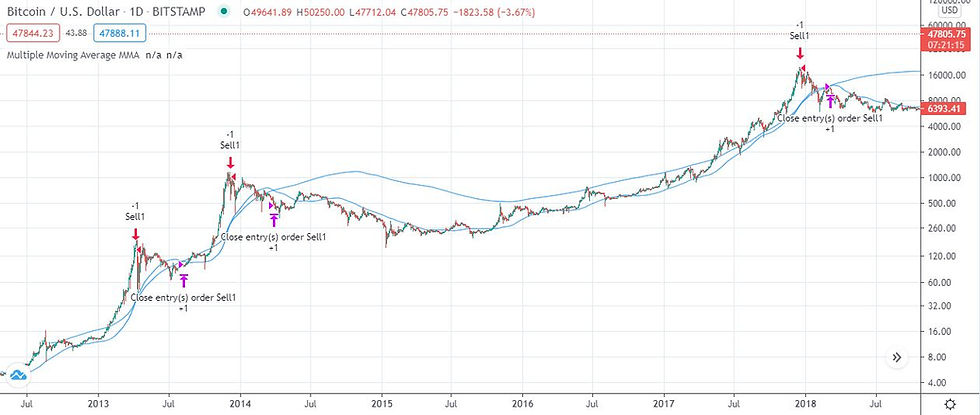top of page
Search
• Dragostin Kozhuharov

# BTC Pi Cycle Top Indicator test

Updated: Mar 29, 2021

The BTC Pi Cycle Top Indicator is an indicator with which I got familiar earlier today. Some friends of mine brought it to my attention as it's supposed to be good at predicting tops for BTC. Furthermore they wanted me to code the indicator in tradingview in order for them to be able to use it.

They send me this link as a guidance: https://www.lookintobitcoin.com/charts/pi-cycle-top-indicator/

## How does it work?

two simple moving averages

first one is 111 day normal simple moving average

second is 350 day multiple moving average. A multiple moving average is calculated when you multiply the price by 2.

When the 111 day moving average crosses over the 350 day multiple moving average, this triggers the sell signal.

There is some more information in the link above how if you divide 350 by 111 the result is very close to Pi, and hence the name of the thing. I don't think this is relevant in any way to the strategy, it's more of a random coincidence, but hey Pi is a great name. I think there was a movie with it in the title as well.

## Multiple Average Indicator Code

But by the time someone sent this link in the group, I had done the code for the Multiple Moving Average indicator. Here it is:

study("Multiple Moving Average MMA", overlay=true)

mom_length = 350

mma = sma(close*2, mom_length)

plot(mma)

## BTC Pi Cycle Top Indicator

I also did my version of the full indicator and here is the code:

study("Multiple Moving Average MMA", overlay=true)

mom_length = 350

sma_length = 111

mma = sma(close*2, mom_length)

sma_1 = sma(close, sma_length)

plot(mma, color = color.red)

plot(sma_1)

When you run this you will see the indicator on the chart with the MMA in red and the SMA in blue.

## The Backtest

After I finished the above, I decided to check how good this indicator is. So I ran a simple backtest with the following parameters - account size = 20,000 , trade size = 1.

A short trade would open when the 111SMA crosses over the 350 MMA. Then the trade would close when the 350MMA crosses over the 111SMA.

I didn't put any SL in, as I wanted to create a quick test and the results showed that one won't be necessary. At least in theory.

This is run on a daily chart.

Here is the code:

strategy("Multiple Moving Average MMA", overlay=true)

mom_length = 350

sma_length = 111

mma = sma(close*2, mom_length)

sma_1 = sma(close, sma_length)

plot(mma)

plot(sma_1)

cross = crossover(sma_1, mma)

closecross = crossover(mma, sma_1)

strategy.entry("Sell1", strategy.short, 1, when = cross)

strategy.close("Sell1", when=closecross)Here are the results from the testWhat we can see is almost no drawdown. All trades are winners and the net profit on a 20k accounts is 8298.32USD or 41.49% return.

It all looks great. But is that the case?

## The Problems

Here are my problems with the above. There isn't enough data to backtest this further and only 3 trades isn't a big enough sample to confirm that this indicator works.

The fact that it works so well raises the question whether some serious data overfitting has happened.

Would I use this strategy?

It doesn't hurt to monitor the indicator and see whether the market sells off when the conditions are met. If I decide to gamble on it, it would be with a close stop loss. But it would be a gamble.